## Animation Quality Metric (AQM)

#### Responses of the AQM for various Pixel Flow velocities. Specular objects with big perceivable differences and holes resulting from the IBR processing are masked out.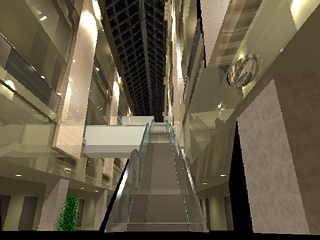The result of 3D warping of keyframe #1.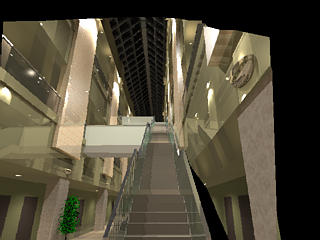The result of 3D warping of keyframe #2.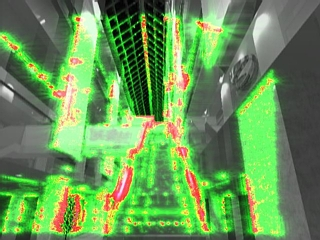It is assumed that Pixel Flow is compensated by the eye tracking motion. P(>0.75) = 11.37% (the percentage of pixels with the probability of detecting perceivable differences over 0.75.)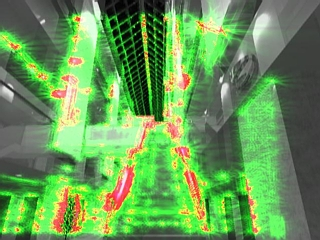It is assumed that the eye does not follow the moving image patterns. The original Pixel Flow was used. P(>0.75) = 10.56%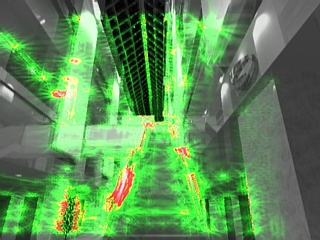It is assumed that the eye does not follow the moving image patterns. The original Pixel Flow was multiplied by the factor 3. P(>0.75) = 3.00%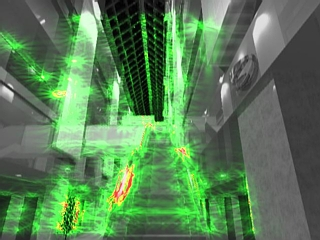It is assumed that the eye does not follow the moving image patterns. The original Pixel Flow was multiplied by the factor 5. P(>0.75) = 0.93%

 Probability of detecting the differences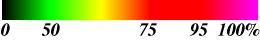Click-on every image to see the actual size and quality of images which were used in our experiment (this requires proper setup of your browser to display images in SGI format). It was assumed that animation is observed from the distance 0.5 meter.